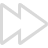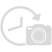# Time-lapse Calculator

Quick and easy way to calculate the time-lapse duration and frame rate.

Share:## Best Time-lapse Calculator

The Time-lapse Calculator provides a quick & easy way to calculate the time-lapse duration and required frames with the memory size required.
You can use this time-lapse calculator for a CCTV time-lapse camera or a time-lapse camera installed on your construction site, agriculture project, or power plant project. This time-lapse calculator is simple to determine the exact time-lapse duration, number of images required, etc. With this calculator, you will never have to spend hours calculating the parameter for your time-lapse.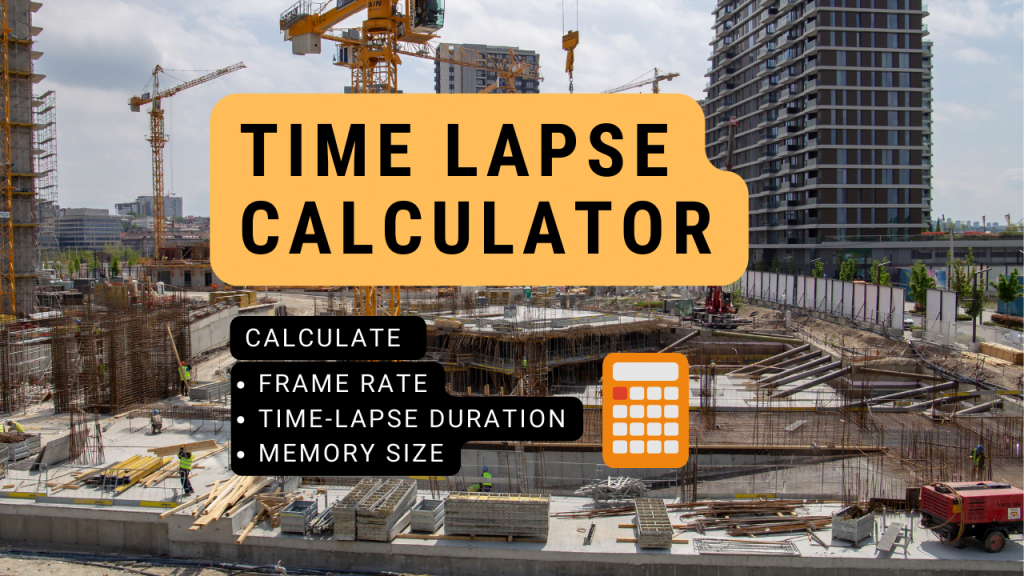### How to Use Our Construction Time-Lapse Calculator?

At the start, you need to select one of the four calculators and follow the below steps.

#### Basic Time-Lapse Duration Calculation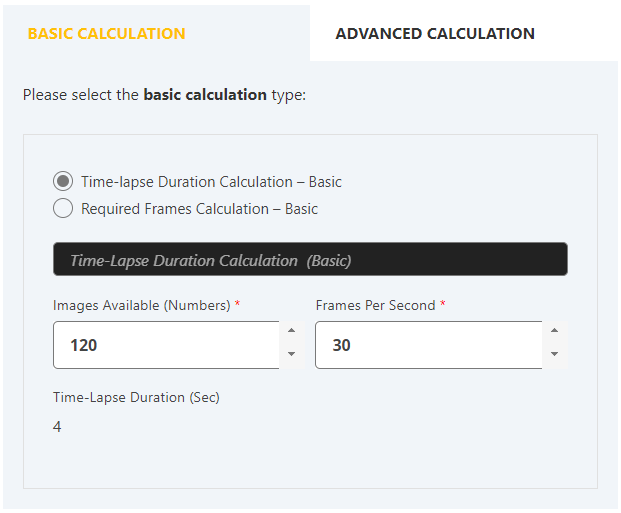To determine the time-lapse duration, you need to enter the number of images available and frames per second (FPS). Our calculator will automatically display the Time-lapse video duration in seconds.

#### Basic Required Frames Calculation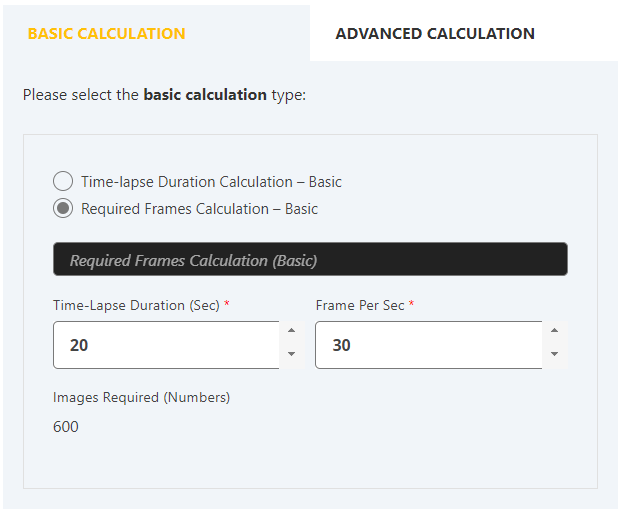Time-lapse duration (sec) and Frame per second (fps) are simple to calculate by entering the total number of images captured.

For long-term time-lapse, the camera or cloud storage may get full. Pre-calculating the memory card size and time-lapse duration can help overcome this problem.With this calculator, you can calculate the number of images, the memory card size required to store captured images, and the time-lapse duration (min). For calculating these, you have to enter the following values

• Shooting Interval (min) ( Ex – one image per 5 min)
• Shooting Hours Per Day
• Shooting Duration (days)
• Frame Per Sec
• Each Image Size (MB)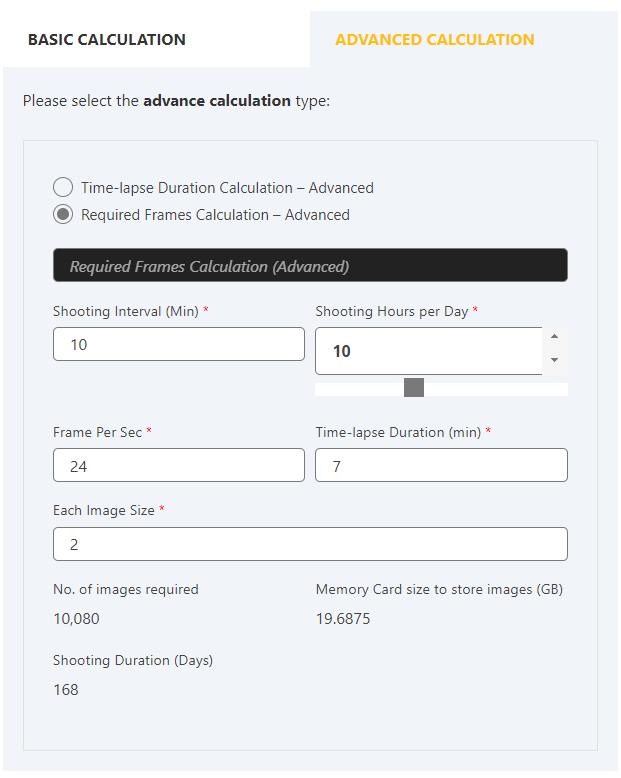With this calculator, you can calculate the number of images, memory card size (GB) required to store the captured images, and the shooting duration (days). For calculating these, you have to enter the following values

• Shooting Interval
• Shooting Hours Per Day
• Time-lapse Duration (min)
• Frame Per Sec
• Each Image Size (MB)

## Time-lapse Calculator Reviews

I am a project manager. I found this calculator helpful. It helped me calculate the memory size required for my project monitoring.

This time-lapse calculator is awesome and simple to use. I have used other time-lapse calculators as well but this is simple and free from ads.

This calculator is helpful for me in determining the number of images and time-lapse duration for our infrastructure project.

### What is a Time Lapse Calculator?

A time-lapse calculator is a custom calculator for computing the clip length, frame rate, number of photos,  and storage usage.

It uses the mathematical equation to determine the days, hours, minutes, or seconds of footage to be compressed in a single few seconds of time-lapse video.

Following are the terms that can be determined with a time-lapse calculator

1. Time-lapse duration
2. Number of images required
3. Memory card size to store images
4. Shooting duration in days

### What Can Our Time-Lapse Calculator Do?

OpticVyu time-lapse calculator can compute four different types of time-lapse calculations.

1. Basic Time-lapse Duration Calculation
2. Basic Required Frames Calculation (Number of images)

Our time-lapse calculator can determine the following parameters of the time-lapse sequence.

Shooting Interval: The shooting interval is the frequency of image capture. For creating a short-term time-lapse video like capturing natural scenery over a day, shooting intervals can be as low as 1 sec. For long-term time-lapse, the image capture interval of 10mins is sufficient.

Shooting Hours: Shooting hours are the number of hours in a day for which a camera actively captures images. For short-term time-lapse, 24 hrs shooting hours are enough to showcase the day & night changes of the subject. Long-term time-lapse (construction of a building) mostly requires only daytime photography.

FPS (Frames Per Second): Frames consumed throughout 1sec of playing a time-lapse is FPS. A higher fps creates a smoother time-lapse. For general purposes, 24 FPS time-lapse is acceptable. However, for short & slow speed time-lapse videos, 10 FPS is best suitable. A cinematic time-lapse video uses 30 or more FPS.

Time-lapse Duration: Depending on the number of images available & desired fps, the time-lapse duration is adjustable. For a catchy time-lapse, keep the time-lapse video duration from 1-2min.

### How to Calculate Time-lapse Duration?

Dividing the number of available images by the frames per second gives the time-lapse duration. For example, if the number of available images is 100 and the required frames per second are 20, then the time-lapse duration will be 5 seconds.